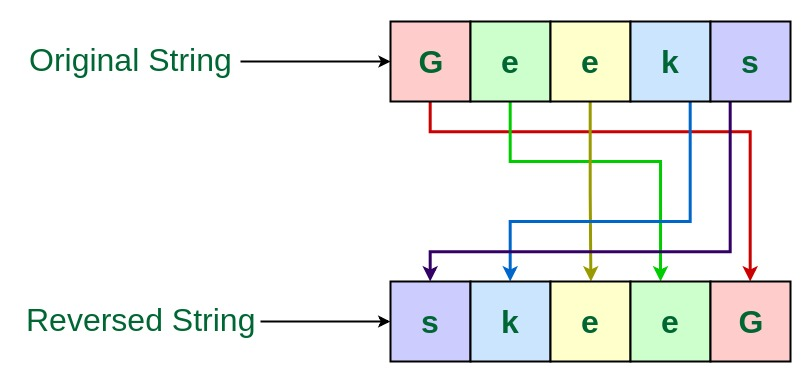Open in App
Not now

# TCS Coding Practice Question | Reverse a String

• Difficulty Level : Medium
• Last Updated : 06 Mar, 2023

Given a string, the task is to reverse this String using Command Line Arguments. Examples:

```Input: Geeks
Output: skeeG

Input: GeeksForGeeks
Output: skeeGroFskeeG```Approach 1: Using another String to store the reverse

• Since the string is entered as Command line Argument, there is no need for a dedicated input line
• Extract the input string from the command line argument
• Create a String to store the resultant reversed string, say reversedString
• Traverse through this String character by character using loop, in reverse order
• Now append each character into the resultant reversedString
• This is the required reversed string

Program:

## C

 `// C program to reverse a string` `// using command line arguments`   `#include ` `#include ` `#include `   `// Function to reverse the String` `char``* reverseString(``char` `input[])` `{`   `    ``// Get the length of the string` `    ``int` `length = ``strlen``(input);` `    ``int` `i;`   `    ``// String to store the reverse` `    ``char``* reversedString` `        ``= (``char``*)``malloc``(length * ``sizeof``(``char``));`   `    ``// Loop through the string` `    ``// character by character in reverse order` `    ``// and store it into the resultant string` `    ``for` `(i = length - 1; i >= 0; i--)` `        ``reversedString[length - 1 - i] = input[i];`   `    ``// Return the reversed String` `    ``return` `reversedString;` `}`   `// Driver code` `int` `main(``int` `argc, ``char``* argv[])` `{` `    ``// Check if the length of args array is 1` `    ``if` `(argc == 1)` `        ``printf``(``"No command line arguments found.\n"``);` `    ``else` `{`   `        ``// Get the command line argument` `        ``// and reverse it` `        ``printf``(``"%s\n"``, reverseString(argv));` `    ``}` `    ``return` `0;` `}`

## Java

 `// Java program to reverse a string` `// using command line arguments`   `class` `GFG {`   `    ``// Function to reverse the String` `    ``public` `static` `String reverseString(String input)` `    ``{`   `        ``// String to store the reverse` `        ``String reversedString = ``""``;`   `        ``// Loop through the string` `        ``// character by character in reverse order` `        ``// and store it into the resultant string` `        ``for` `(``int` `i = input.length() - ``1``; i >= ``0``; i--)` `            ``reversedString += input.charAt(i);`   `        ``// Return the reversed String` `        ``return` `reversedString;` `    ``}`   `    ``// Driver code` `    ``public` `static` `void` `main(String[] args)` `    ``{`   `        ``// Check if length of args array is` `        ``// greater than 0` `        ``if` `(args.length > ``0``) {`   `            ``// Get the command line argument` `            ``// and reverse it` `            ``System.out.println(reverseString(args[``0``]));` `        ``}` `        ``else` `            ``System.out.println(``"No command line "` `                               ``+ ``"arguments found."``);` `    ``}` `}`

Output:

Approach 2: Without using another String to store the reverse

• Since the string is entered as Command line Argument, there is no need for a dedicated input line
• Extract the input string from the command line argument
• Traverse through this String character by character using loop till half the length of the string
• Swap the characters from one end with the characters from the other end
• This is the required reversed string

Program:

## C

 `// C program to reverse a string` `// using command line arguments`   `#include ` `#include ` `#include `   `// Function to reverse a string` `char``* reverseString(``char` `str[])` `{`   `    ``int` `n = ``strlen``(str);` `    ``int` `i;` `    ``char` `temp;`   `    ``// Swap character starting from two` `    ``// corners` `    ``for` `(i = 0; i < n / 2; i++) {` `        ``temp = str[i];` `        ``str[i] = str[n - i - 1];` `        ``str[n - i - 1] = temp;` `    ``}`   `    ``return` `str;` `}`   `// Driver code` `int` `main(``int` `argc, ``char``* argv[])` `{` `    ``// Check if the length of args array is 1` `    ``if` `(argc == 1)` `        ``printf``(``"No command line arguments found.\n"``);` `    ``else` `{`   `        ``// Get the command line argument` `        ``// and reverse it` `        ``reverseString(argv);`   `        ``// Print the reversed string` `        ``printf``(``"%s\n"``, argv);` `    ``}` `    ``return` `0;` `}`

## Java

 `// Java program to reverse a string` `// using command line arguments`   `class` `GFG {`   `    ``// Function to reverse the String` `    ``public` `static` `String reverseString(String str)` `    ``{`   `        ``int` `n = str.length();` `        ``char` `temp;`   `        ``// Swap character starting from two` `        ``// corners` `        ``for` `(``int` `i = ``0``; i < n / ``2``; i++) {` `            ``str = str.substring(``0``, i)` `                  ``+ str.charAt(n - i - ``1``)` `                  ``+ str.substring(i + ``1``, n - i - ``1``)` `                  ``+ str.charAt(i)` `                  ``+ str.substring(n - i);` `        ``}`   `        ``return` `str;` `    ``}`   `    ``// Driver code` `    ``public` `static` `void` `main(String[] args)` `    ``{`   `        ``// Check if length of args array is` `        ``// greater than 0` `        ``if` `(args.length > ``0``) {`   `            ``// Get the command line argument` `            ``// and reverse it` `            ``System.out.println(reverseString(args[``0``]));` `        ``}` `        ``else` `            ``System.out.println(``"No command line "` `                               ``+ ``"arguments found."``);` `    ``}` `}`

Output:

My Personal Notes arrow_drop_up
Related Articles